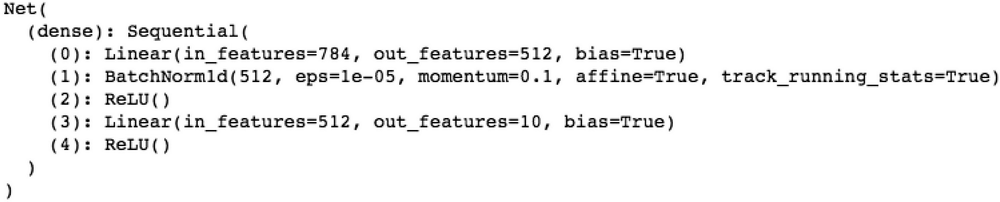# AI 安全之对抗样本入门 (35)：打造对抗样本工具箱 3.4(PyTorch)

PyTorch 是 torch 的 Python 版本，是由 Facebook 开源的神经网络框架。PyTorch 虽然是深度学习框架中的后起之秀，但是发展极其迅猛。PyTorch 提供了 NumPy 风格的 Tensor 操作，熟悉 NumPy 操作的用户非常容易上手。我们以解决经典的手写数字识别的问题为例，介绍 PyTorch 的基本使用方法，代码路径为：

https://github.com/duoergun0729/adversarial_examples/blob/master/code/2-pytorch.ipynb
1. 加载相关库

import osimport torchimport torchvisionfrom torch.autograd import Variableimport torch.utils.data.dataloader as Data
1. 加载数据集

PyTorch 中针对常见的数据集进行了封装，免去了用户手工下载的过程并简化了预处理的过程。这里需要特别指出的是，PyTorch 中每个 Tensor 包括输入节点，并且都可以有自己的梯度值，因此训练数据集要设置为 train=True，测试数据集要设置为 train=False：

train_data = torchvision.datasets.MNIST( 'dataset/mnist-pytorch', train=True,transform=torchvision.transforms.ToTensor(), download=True)test_data = torchvision.datasets.MNIST( 'dataset/mnist-pytorch', train=False,transform=torchvision.transforms.ToTensor())

transform=transforms.Compose([torchvision.transforms.ToTensor(),                 torchvision.transforms.Normalize([0.5], [0.5])])

Downloading http://yann.lecun.com/exdb/mnist/train-images-idx3-ubyte.gzDownloading http://yann.lecun.com/exdb/mnist/train-labels-idx1-ubyte.gzDownloading http://yann.lecun.com/exdb/mnist/t10k-images-idx3-ubyte.gzDownloading http://yann.lecun.com/exdb/mnist/t10k-labels-idx1-ubyte.gzProcessing... Done!
1. 定义网络结构

class Net(torch.nn.Module):    def __init__(self):        super(Net, self).__init__()        self.dense = torch.nn.Sequential(            #全连接层            torch.nn.Linear(784, 512),            #BatchNorm 层            torch.nn.BatchNorm1d(512),            torch.nn.ReLU(),            torch.nn.Linear(512, 10),            torch.nn.ReLU()        )    def forward(self, x):        #把输出转换成大小为 784 的一维向量        x = x.view(-1, 784)        x=self.dense(x)        return torch.nn.functional.log_softmax(x, dim=1)1. 定义损失函数和优化器

optimizer = torch.optim.Adam(model.parameters())loss_func = torch.nn.CrossEntropyLoss()
1. 训练与验证

PyTorch 的训练和验证过程是分开的，在训练阶段需要把训练数据进行前向传播后，使用损失函数计算训练数据的真实标签与预测标签之间损失值，然后显示调用反向传递 backward()，使用优化器来调整参数，这一操作需要调用 optimizer.step()：

for i, data in enumerate(train_loader):            inputs, labels = data            inputs, labels = inputs.to(device), labels.to(device)            # 梯度清零            optimizer.zero_grad()            # 前向传播            outputs = model(inputs)            loss = loss_func(outputs, labels)            #反向传递            loss.backward()            optimizer.step()

# 每训练 100 个批次打印一次平均损失值sum_loss += loss.item()if (i+1) % 100 == 0:   print('epoch=%d, batch=%d loss: %.04f'% (epoch + 1, i+1, sum_loss / 100))        sum_loss = 0.0

# 每跑完一次 epoch，测试一下准确率进入测试模式，禁止梯度传递with torch.no_grad():     correct = 0     total = 0     for data in test_loader:                images, labels = data                images, labels = images.to(device), labels.to(device)                outputs = model(images)                # 取得分最高的那个类                _, predicted = torch.max(outputs.data, 1)                total += labels.size(0)                correct += (predicted == labels).sum()                print('epoch=%d accuracy=%.02f%%' % (epoch + 1, (100 * correct /                total)))

epoch=20, batch=100 loss: 0.0035epoch=20, batch=200 loss: 0.0049epoch=20, batch=300 loss: 0.0040epoch=20, batch=400 loss: 0.0042epoch=20 accuracy=97.00%

PyTorch 保存的模型文件后缀为 pth：

torch.save(model.state_dict(), 'models/pytorch-mnist.pth')